# Constructing a Copy of Line Segment

Geometry is an ancient science and an important branch of mathematics. Every day we come across many shapes in daily life, we call them geometrical shapes. The idea of construction came from these shapes.  Consider a triangle, a rectangle, a square or any other shape. Every shape begins with a point and then extends to be a line or a line segment or a curve. Number and arrangement of these line segments decide the type of figure. To construct any geometrical figure we need to have an idea to construct a line segment. Let’s learn how to construct a replica of a given line segment.

## Construction in Geometry

In geometry, construction means drawing lines, shapes or angles accurately using pencils, rulers and compasses. This is the pure geometric construction i.e. no measurements, no numbers. So let’s learn pure geometric constructions of a line segments step by step.

A point is a small dot which is the starting point of a line segment. By definition, a line segment is a part of a line which connects two points within a line. Different numbers of line segments give us different figures and such figures may be either open figure or closed figures. A line segment is denoted by its two endpoints. In the figure, m is a line and AB is the line segment on the line m.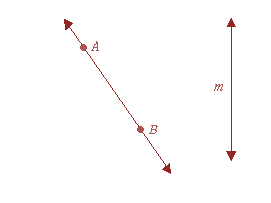### Copy of Line Segments Construction Procedure

We can construct a line segment of a given length either by using a ruler or a combination of ruler and compass. Construction of a copy of a given line segment can also be achieved easily by using either of the above methods. However, the construction of line segments is not that accurate when we use a ruler only. Hence, a ruler-compass combination is always a better approach to construct a copy of line segments. The construction for the copy of the line segment has a  place to begin the copy It is necessary to draw the straight line for the given measure in order to start the procedure for copying. Such a kind of line is called the reference line.

Steps for Construction:

Let’s construct a copy of a line segment XY using ruler and compass together.

Step 1: Let be a line segment of unknown length.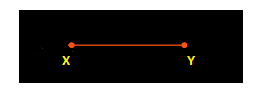Step 2: Fix the compass’ pointer on the point X and pencil pointer on Y gives you the length of XY.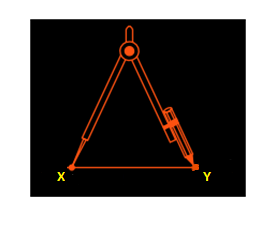Step 3: Now draw a straight line using a ruler, let’s say line m.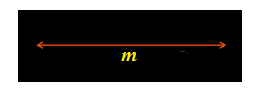Step 4: Mark a point A and fix the compass pointer (step 2)on A without changing the settings.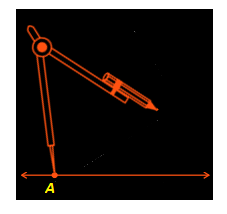Step 5: Fixing compass on A draw an arc on, name the point at which pencil pointer cuts as B.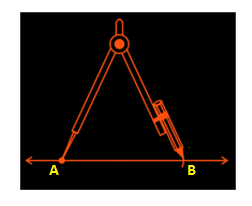Now is a copy of i.e. length of AB will same as that of XY (if you didn’t change the compass settings).

### Proof for the Copy of Line Segments

Here, the compass is considered as a measuring tool for the purpose of copying the line segment. Since the original line segment and the copied line segment are of the same measure, the segments are considered as congruent.

For more about geometric constructions and to watch interactive videos on the topic, download BYJU’S – The Learning App. Also, take free tests to practice for exams.